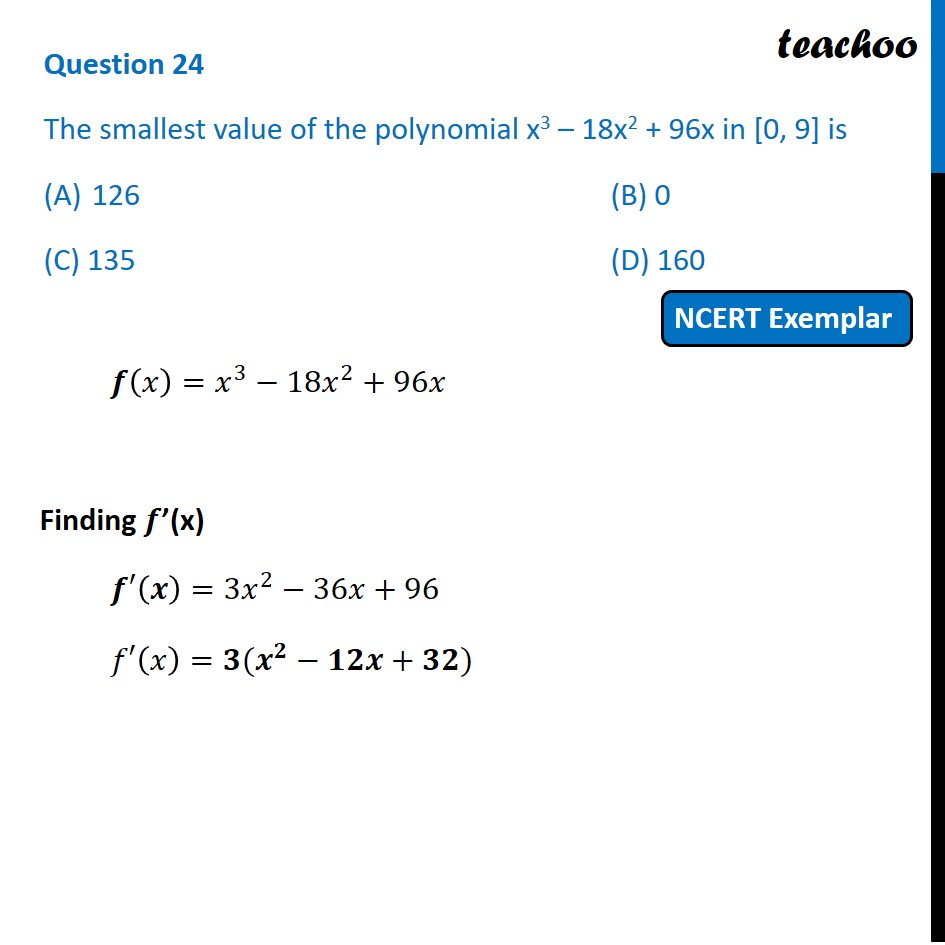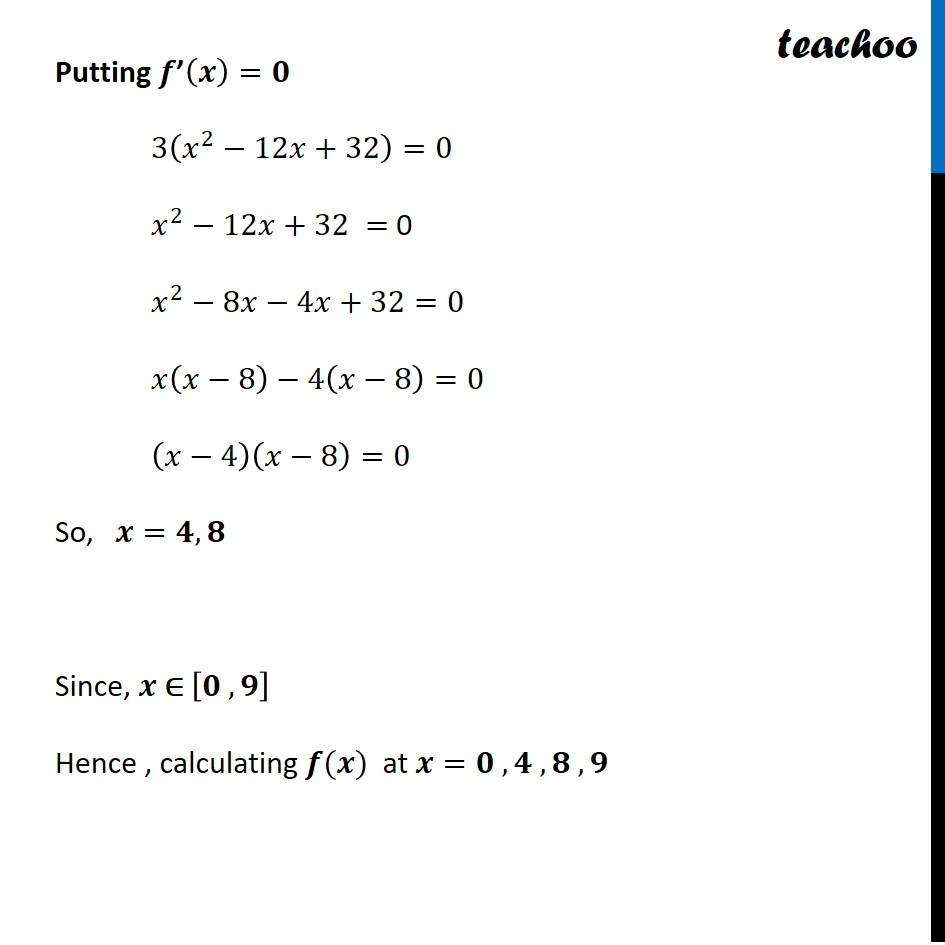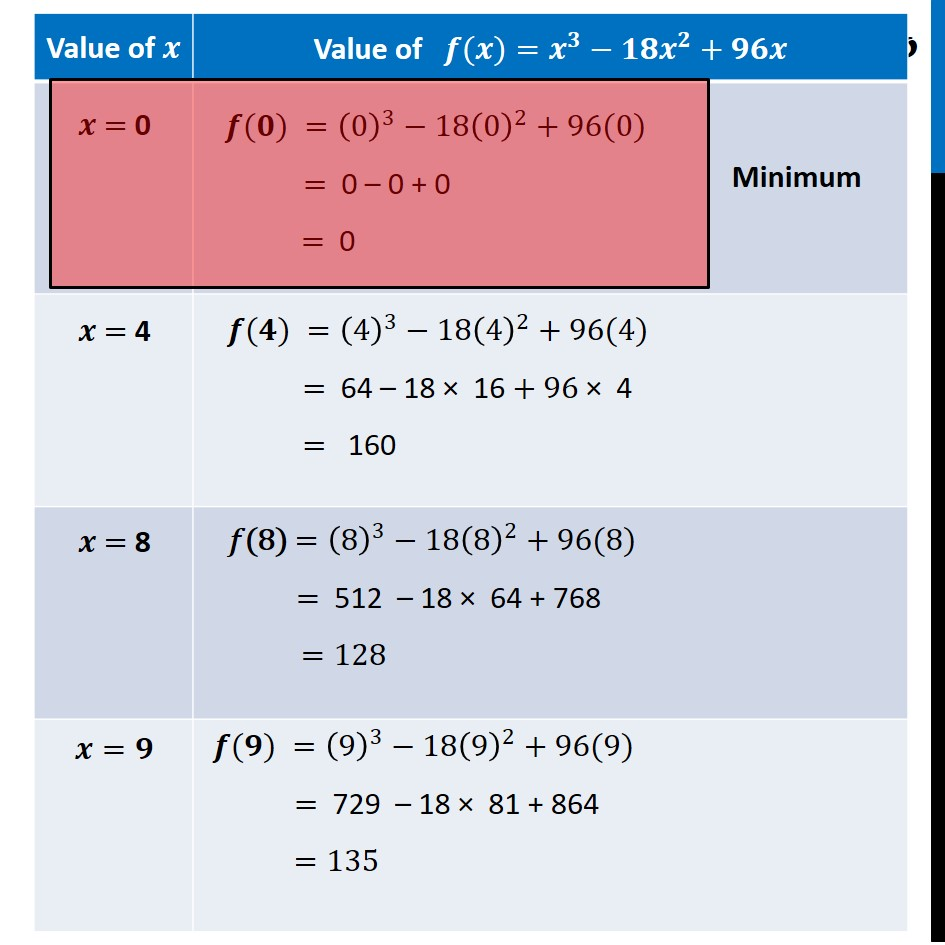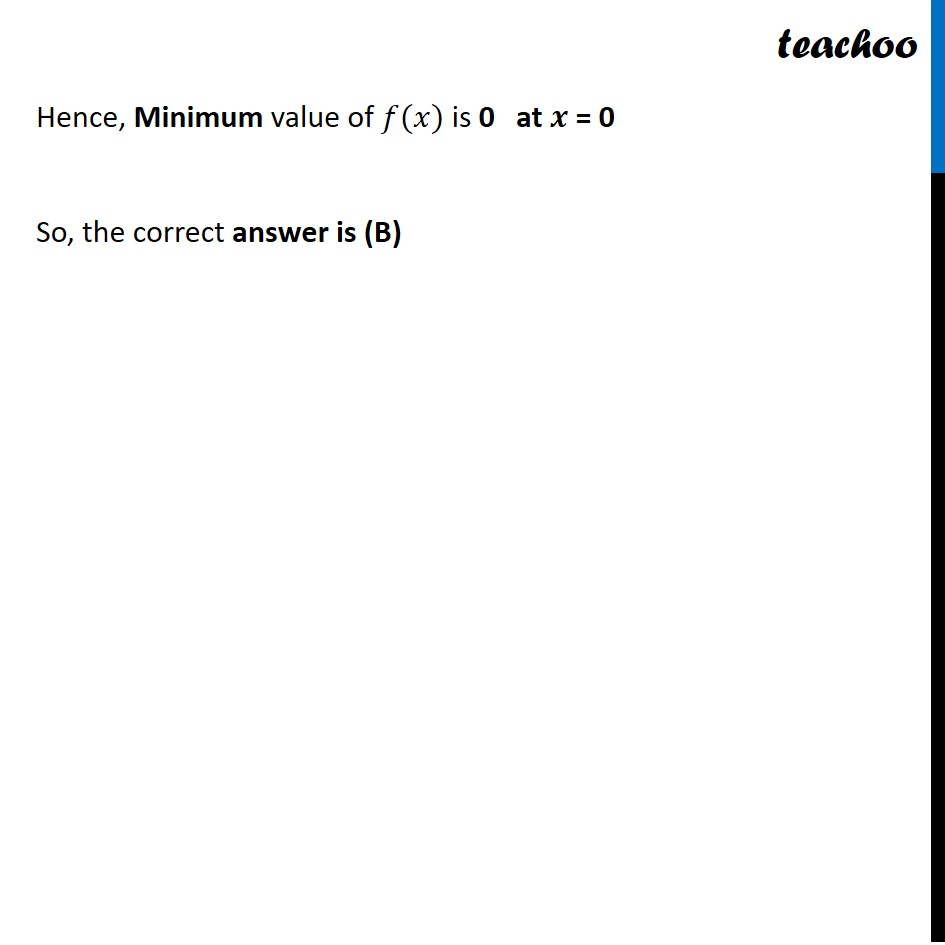NCERT Exemplar - MCQs

Chapter 6 Class 12 Application of Derivatives
Serial order wise

## (C) 135                    (D) 160

This question is similar to Ex 6.5, 7 - Chapter 6 Class 12 - Application of DerivativesLearn in your speed, with individual attention - Teachoo Maths 1-on-1 Class

### Transcript

Question 11 The smallest value of the polynomial x3 – 18x2 + 96x in [0, 9] is 126 (B) 0 (C) 135 (D) 160 𝒇(𝑥)=𝑥^3−18𝑥^2+96𝑥 Finding 𝒇’(x) 𝒇′(𝒙)=〖3𝑥〗^2−36𝑥+96 𝑓′(𝑥)=𝟑(𝒙^𝟐−𝟏𝟐𝒙+𝟑𝟐) Putting 𝒇’(𝒙)=𝟎 3(𝑥^2−12𝑥+32)=0 𝑥^2−12𝑥+32 = 0 𝑥^2−8𝑥−4𝑥+32=0 𝑥(𝑥−8)−4(𝑥−8)=0 (𝑥−4)(𝑥−8)=0 So, 𝒙=𝟒, 𝟖 Since, 𝒙 ∈ [𝟎 , 𝟗] Hence , calculating 𝒇(𝒙) at 𝒙=𝟎 , 𝟒 , 𝟖 , 𝟗 𝒇(𝟒) =(4)^3−18(4)^2+96(4) = 64 – 18 × 16 + 96 × 4 = 160 𝑓(8) =(8)^3−18(8)^2+96(8) = 512 – 18 × 64 + 768 =128 𝒇(𝟗) =(9)^3−18(9)^2+96(9) = 729 – 18 × 81 + 864 =135 Hence, Minimum value of 𝑓(𝑥) is 0 at 𝒙 = 0 So, the correct answer is (B)